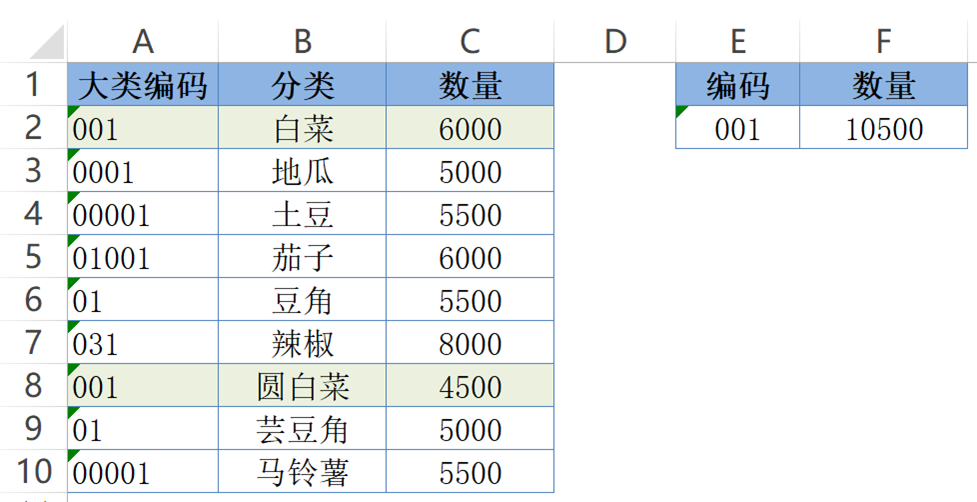# SUMPRODUCT：没有人比我更懂求和

F2单元格公式为：
=SUMPRODUCT((A2:A13=E2)*C2:C13)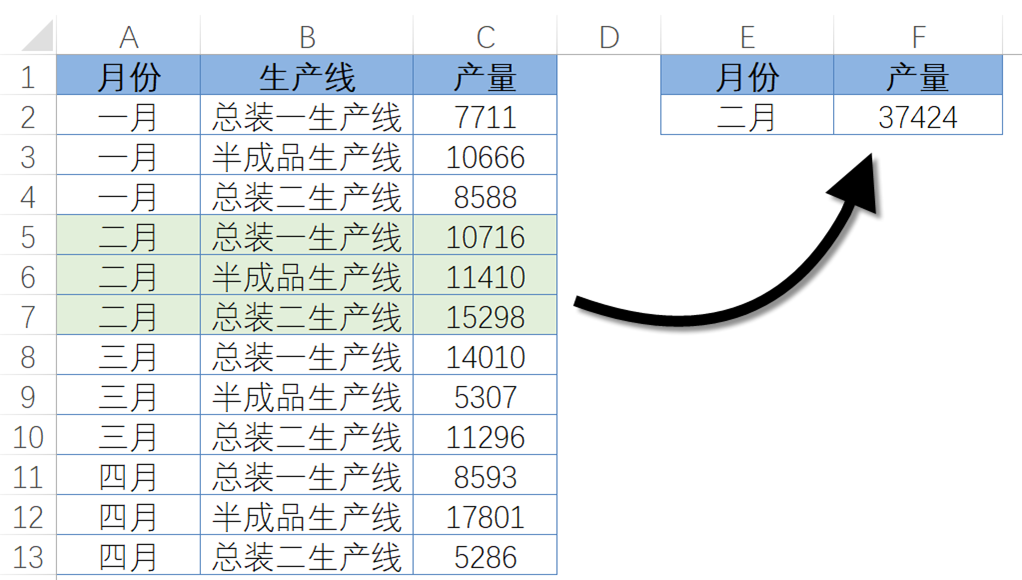E2单元格公式为：
=SUMPRODUCT(ISNUMBER(FIND(“华为”,B2:B10))*C2:C10)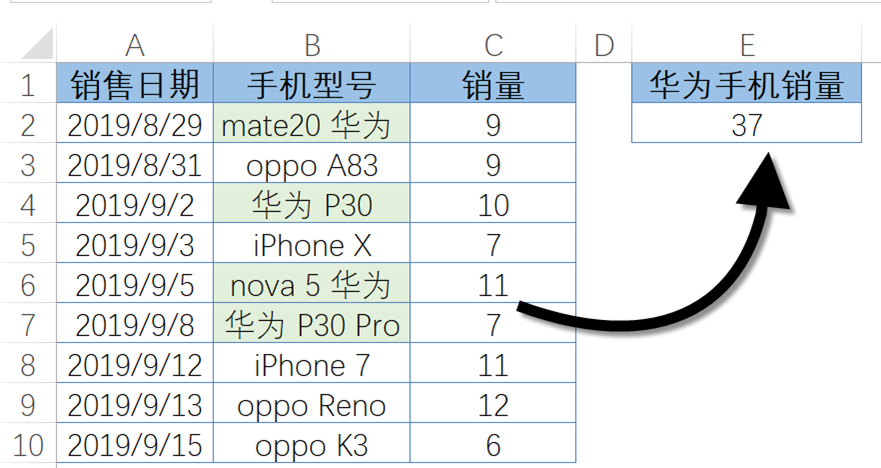F2输入以下公式下拉：
=SUMPRODUCT((MONTH(A\$2:A\$11)=E2)*C\$2:C\$11)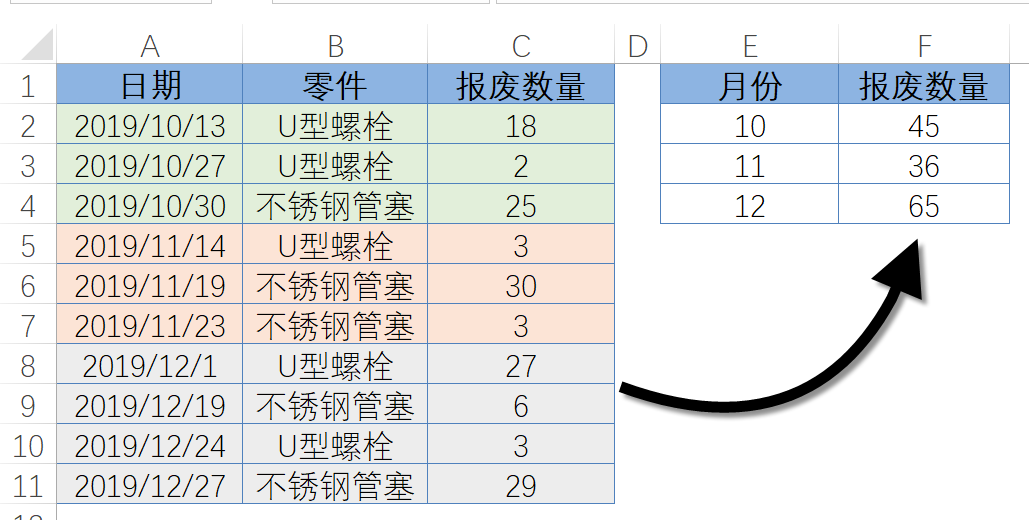H2单元格公式为：
=SUMPRODUCT((B2:B10=G2)*C2:E10)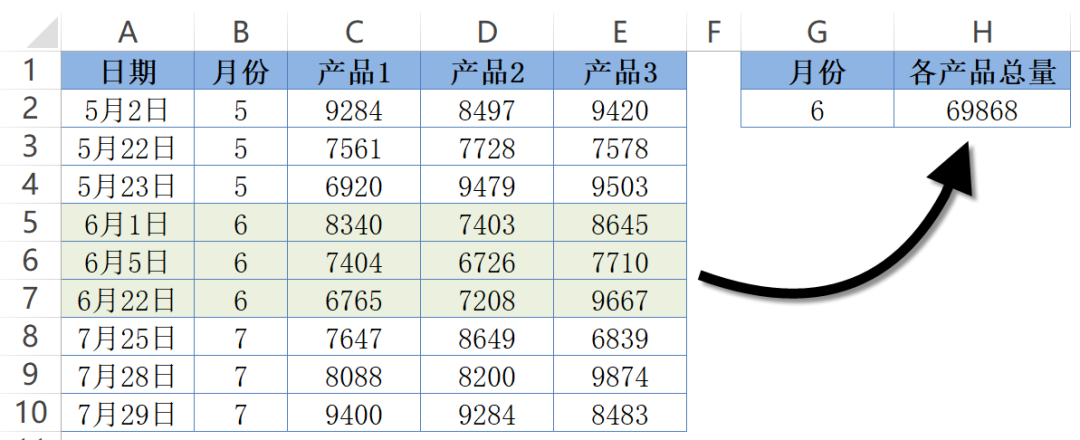F2单元格公式为：
=SUMPRODUCT((A2:A10=E2)*C2:C10)